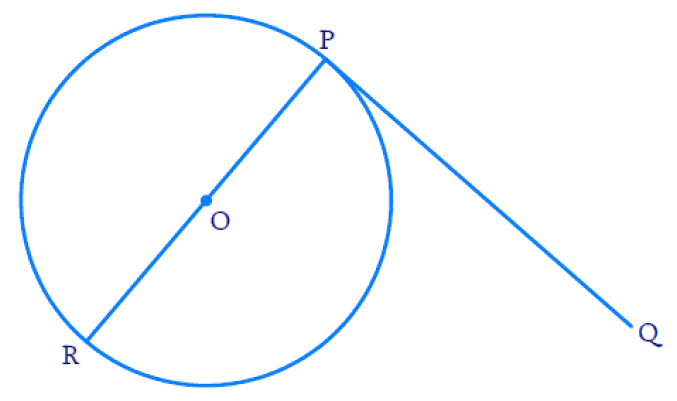# Ex.10.2 Q5 Circles Solution - NCERT Maths Class 10

Go back to  'Ex.10.2'

## Question

Prove that the perpendicular at the point of contact to the tangent to a circle passes through the center.

Video Solution
Circles
Ex 10.2 | Question 5

## Text Solution

To prove:

Perpendicular at the point of contact to the tangent to a circle passes through the centre.

Reasoning and Steps:By theorem, tangent at any point of a circle is perpendicular to the radius through the point of contact.

Radius will always pass through the center of the circle.

Hence proved that perpendicular $$PR$$ of tangent $$PQ$$ passes through center $$O.$$

Learn from the best math teachers and top your exams

• Live one on one classroom and doubt clearing
• Practice worksheets in and after class for conceptual clarity
• Personalized curriculum to keep up with school